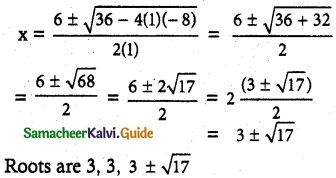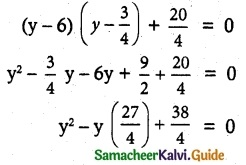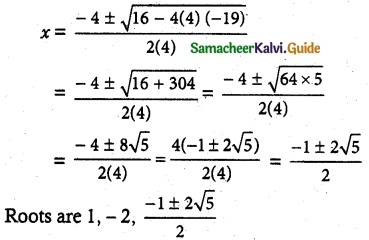Tamilnadu State Board New Syllabus Samacheer Kalvi 12th Maths Guide Pdf Chapter 3 Theory of Equations Ex 3.4 Textbook Questions and Answers, Notes.

## Tamilnadu Samacheer Kalvi 12th Maths Solutions Chapter 3 Theory of Equations Ex 3.4

Question 1.
Solve:
(i) (x – 5) (x – 7) (x + 6)(x + 4) = 504
Solution:
(x – 5) (x + 4) (x – 7) (x + 6) = 504
(x2 – x – 20) (x2 – x – 42) = 504
Let y = (x2 – x)
(y – 20) (y – 42) = 504
⇒ y2 – 42y – 20y + 840 = 504
⇒ y2 – 62y + 336 = 0
⇒ (y – 56) (y – 6) = 0
⇒ (y – 56) = 0 or (y – 6) = 0
⇒ x2 – x – 56 = 0 or x2 – x – 6 = 0
⇒ (x – 8) (x + 7) = 0 or (x – 3) (x + 2) = 0
⇒ x = 8, -7 or x = 3, -2
The roots are 8, -7, 3, -2

(ii) (x – 4)(x – 2)(x- 7)(x + 1) = 16
Solution:
(x – 4) (x – 7) (x – 2) (x + 1) = 16
⇒ (x – 4) (x – 2) (x – 7) (x + 1) = 16
⇒ (x2 – 6x + 8) (x2 – 6x – 7) = 16
Let x2 – 6x = y
(y + 8)(y – 7) = 16
⇒ y2 – 7y + 8y – 56 – 16 = 0
⇒ y2 + y – 72 = 0
⇒ (y + 9) (y – 8) = 0
y + 9 = 0
x2 – 6x + 9 = 0
(x – 3)2 = 0
x = 3, 3
or
y – 8 = 0
x2 – 6x – 8 = 0Question 2.
Solve:
(2x – 1)(x + 3)(x – 2)(2x + 3) + 20 = 0
Solution:
(x + 3)(x – 2) (2x – 1)(2x + 3) + 20 = 0
(x² + x – 6) (4x² + 6x – 2x – 3) + 20 = 0
(x² + x – 6) (4x² + 4x – 3) + 20 = 0
(x² + x – 6) (x² + 4x – $$\frac{3}{4}$$) + 20 = 0
(÷4) (x² + x – 6) (x² + x – $$\frac{3}{4}$$) + $$\frac{20}{4}$$ = 0
Put y = x² + x4y² – 27y – 38 = 0
4y² – 8y – 19y + 38 = 0
(4y – 19) (y – 2) = 0
y = 2 (or) y = $$\frac{19}{4}$$
x² + x = 2 (or) x² + x = $$\frac{19}{4}$$
x² + x – 2 = 0 (or) 4x² + 4x = 19
(x + 2)(x – 1) = 0 (or) 4x² + 4x – 19 = 0
x = -2 or x = 1